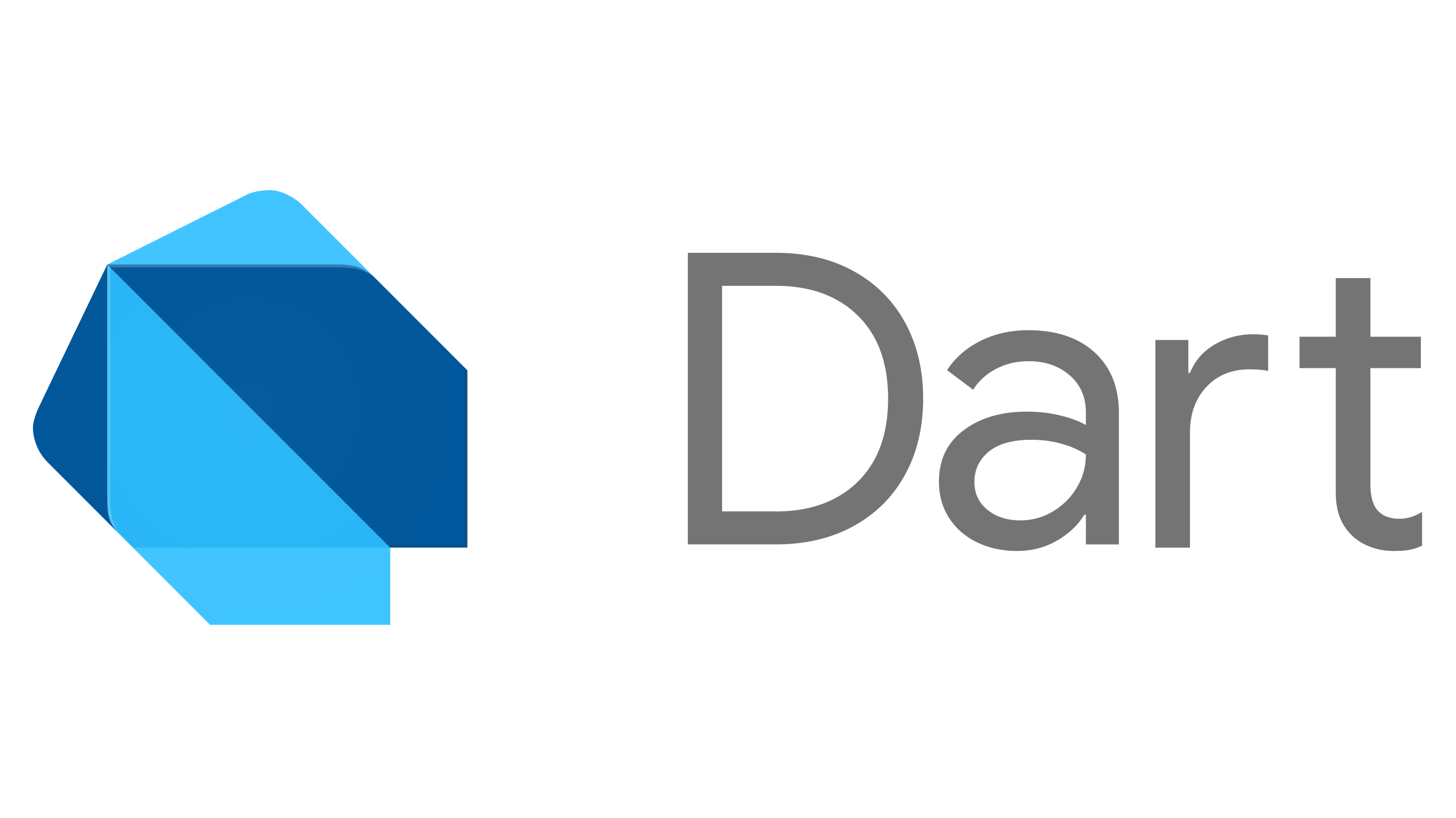Nitish Kumar Singh8 minutes
Posted on
February 21, 2019

# var vs dynamic in Dart

In Dart and JavaScript we have var keyword for the variable declaration but both are not same.

Dart var keyword is very much similar to javascript var keyword but not the exact same. Most of Dart developer uses Dart var as JS var which is not correct. Let’s understand this by some examples.

There is the dynamic keyword for variable declaration in Dart which is equivalent to JavaScript var keyword.

I will give 4 different examples.

# Example 1 #

In JavaScript and Dart, we can store any kind of data in a variable which has been defined using var keyword.

Dart Code Examplemain.dart
``````// This is valid Example this will work
void main() {
var x = 'Maths pi';
var y = 3.14;

print(x); // Maths pi
print(y); // 3.14
}
``````

JavaScript code Examplescript.js
``````// This is valid Example this will work
var x = 'Maths pi';
var y = 3.14;

console.log(x); // Maths pi
console.log(y); // 3.14
``````

# Example 2 #

In JavaScript and Dart, we can store different kind of data in the same variable.

Dart Code Examplemain.dart
``````// This is valid Example this will work
void main() {
var x ;
x = 'Math pi';
print(x); // Maths pi

x = 3.14;
print(x); // 3.14
}
``````

JavaScript code Examplescript.js
``````// This is valid Example this will work
var x ;
x = 'Math pi';
console.log(x); // Maths pi

x = 3.14;
console.log(x); // 3.14
``````

# Example 3 #

Now I am going to mix Example 1 and Example 2 Now I am going to initialize the variable and trying to store different data type in the same variable. This is going to give me some error because this is not valid in Dart but its valid in JavaScript.

This is the place where `var` behaves differently in Dart

Dart Code Examplemain.dart
``````// This is invalid Example and this will not work
void main() {

var x = 'Math pi';
print(x);  // Maths pi

x = 3.14;  // Compilation failed error because
// you are trying to assign double to String variable.
print(x);
}
``````

JavaScript code Examplescript.js
``````// This is valid Example this will work
var x ;
x = 'Math pi';
console.log(x); // Maths pi
x = 3.14;
console.log(x); // 3.14
``````

# Example 4 #

If we use dynamic instead of var in Dart code, We’ll not get any error. Dart dynamic is same as JavaScript var.main.dart
``````// This is valid Example, this will work
void main() {
dynamic x = 'Math pi';
print(x); // Maths pi
x = 3.14;
print(x); // 3.14
}
``````

JavaScript code Examplescript.js
``````// This is valid Example this will work
var x ;
x = 'Math pi';
console.log(x); // Maths pi
x = 3.14;
console.log(x); // 3.14
``````

# Thanks #

Last updated at Tuesday, Jun 9, 2020 by Nitish Kumar Singh